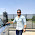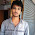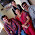# Solved examples of number series in Quantitative aptitude

As we know, questions related to number series are very important in Quantitative aptitude section, So, today I'm going to discuss some problems of number series. These are just for your practice. I have already discussed this chapter in previous session i.e. Sequence and Series. Read this article first, then go through these examples.

## Examples with Solutions

Example1: In the following given series, find out the wrong number.

1, 3, 10, 21, 64, 129, 356

Solution: As i have discussed in previous session, operations applied on series can be: Addition or subtraction, multiplication or division, squaring or cubing and combination of any.

Series                 1        3       10       21      64       129          356
Difference               2        7       11       43       65         227

By studying the above series, you'll come to know that, this is a combination of operations. Operation used is discussed as follows:

Alternately using: (times 2 + 1) and (times 3 + 1)

1 times 2 + 1 = 3
3 times 3 + 1 = 10
10 times 2 + 1 = 21
21 times 3 + 1 = 64
64 times 2 + 1 = 129
129 times 3 + 1 = 388

Therefore, last number of series is wrong i.e.356

Example2: Find the next number of the following series:

672, 560, 448, 336, 224,?

Solution: Check out the difference of each number first:

Series:           672      560     448     336     224
Difference:         112      112      112    112

Difference between all the terms is same i.e. 112

Therefore, next term will be = 224 - 112 = 112 Ans

Example3: Find the next term of following series:
82, 67, 54, 43, 34

Solution: The operation used in series is discussed as follow:
9^2 + 1 = 82
8^2 + 3 = 67
7^2 + 5 = 54
6^2 + 7 = 43
5^2 + 9 = 34

Continuing like this;

4^2 + 11 = 27

Therefore, 27 is the next term.

Example4: What should come in place of question mark?

1721, 2190, 2737, 3368,?

Solution: As we can see, first term is approximately equal to the cube of 12, so firstly we will try to solve it with the cubes.

(12)^3 - 7 = 1721
(13)^3 - 7 = 2190
(14)^3 - 7 = 2737
(15)^3 - 7 = 3368

Now you can find, that there is some pattern. So, continuing like this, we get
(16)^3 - 7 = 4089 Ans

Example5: Find the next term of following series:

8, 24, 12, 36, 18, ?

Solution: Relation between the terms is as follows:

8 times 3= 24

(24/2) = 12

12 times 3=36

(36)/2=18

Continuing like this:
18 times 3 = 54

Therefore, next term is 54

Example 6: Find the next term of the following series:

47, 33, 21, 11, ?

Solution: The pattern used in the series is as follows:

7^2 - 2 = 47
6^2 - 3 = 33
5^2 - 4 = 21
4^2 - 5 = 11
By continuing, we get
3^2 - 6 = 3
Therefore, 3 is the next term.

I will soon update the questions for your practice.

#### What's trending in BankExamsToday

Smart Prep Kit for Banking Exams by Ramandeep Singh - Download here

##### Join 40,000+ readers and get free notes in your email#### 13 comments:

1.Nice 1... Really Healpful

2.3.4.5.what is next number in this series 11 19 13 21 17 22 25

1.2.3.4.answer is 22
xx , 19 , xx , 19+2=21 , xx , 21+1=22 , xx , [22+0=22]

6.1,7,12,?,45,147 with solution

7.8.9.Thank you....

I will try to respond asap